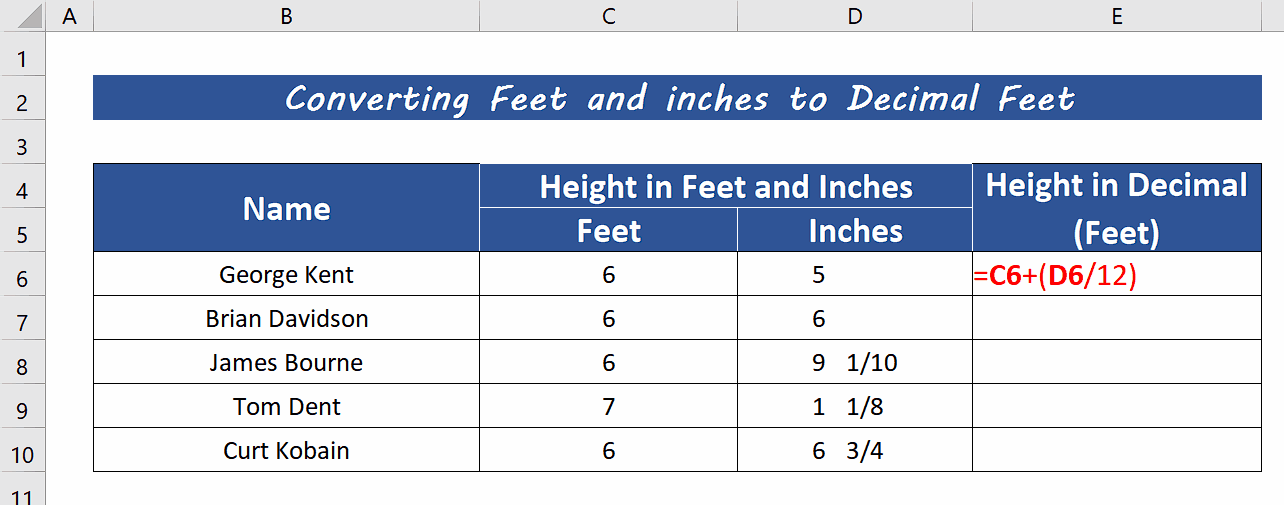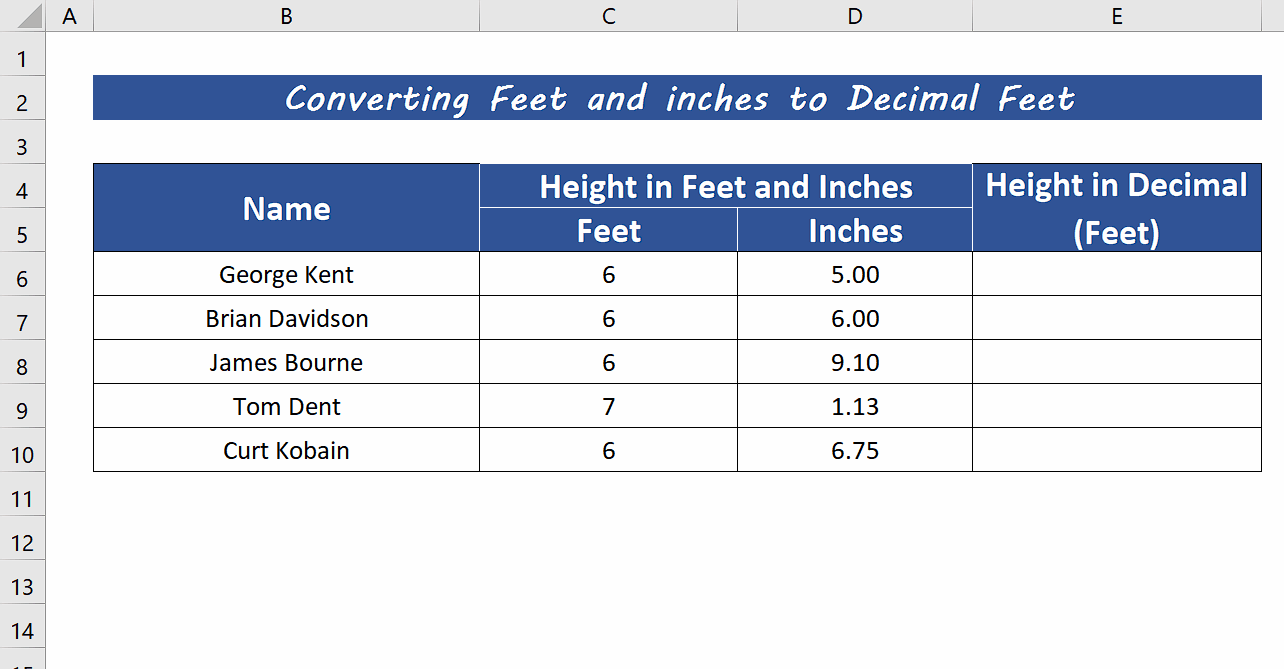# How to convert feet and inches to decimal in Excel

So, in this tutorial we’ll learn how to convert feet and inches measurement to decimal using Excel. We need to understand the conversion formula of Feet and inches to decimal Feet first. Let’s look at the following dataset, where we have feet and inches already in different cells. In this case, we need to convert the inches to decimal only and then combine both whole number feet and decimal inches together. Let’s do this by following the steps mentioned below;There are a few reasons why you might need to convert feet and inches to decimal. For example, some people use feet and inches to measure height, and others use decimals to express height. If you need to compare the height of two people and one person is using feet and inches and the other is using decimal, you will need to convert the measurements to the same unit in order to compare them accurately.

### Step 1 – Convert the fraction format to decimal inches– Our dataset shows the inches in decimal format and we wish to convert them to a fraction of inches to understand the data in a better way. So, select all the column with decimal inches and press CTRL+1.

– This will open up the Format Cells dialog box.

– In the Category field click on Fraction.

– In the Type: field choose Up to two digits format and click on the OK button.

– This will convert the decimal inches to a more understandable format of fractions of inches as shown above.

### Step 2 – Create the formula to convert Feet and inches to decimal feet– We’ll convert the inches to the decimal portion first and for that purpose, we’ll use the following formula =C6 + (D6 / 12).

– This formula will convert the inches to decimal feet first and then add the whole number Feet to it. This will finally give us the Feet in decimal format, as shown above.